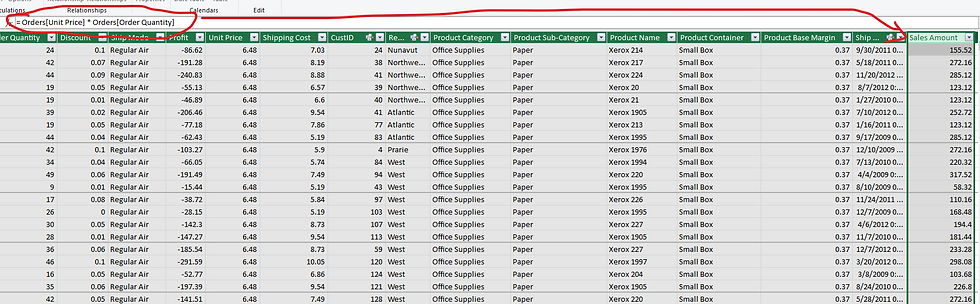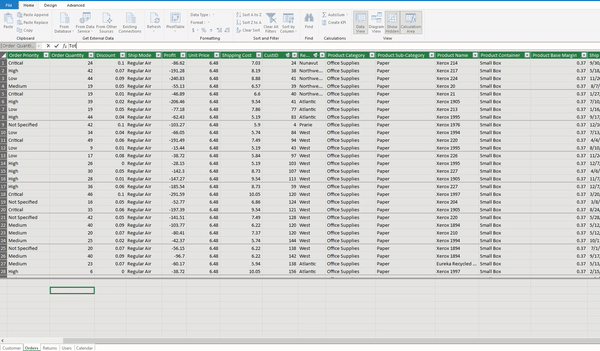top of page
Search

# Say Hello to DAX!In the previous article, we learn to connect, transform, and build relationships between the data tables. We also know how to create a simple Pivot report by combining different facts (numbers) and dimensions (categories).

## What is DAX?

DAX, which stands for Data Analysis eXpressions, is the programming language of Microsoft Analysis Services, Power BI and Excel Power Pivot. It was created in 2010, with the first release of Power Pivot for Excel 2010.

DAX is a functional language. Every expression in DAX is a function call. Something like Excel functions. In Excel and DAX, the concepts of statements, loops, and jumps do not exist as compared to other programming languages.

The Excel users may find some of the DAX expressions familiar. The major shift between Excel functions and DAX is that Excel performs calculations over cells. In Excel, we can reference a cell using its coordinates. For example, we can write the formula:

``` =A1 + (B1 * 2)
```

DAX works on tables and columns, not cells; hence DAX expressions refer to tables and columns. It is something like how the formula works in the Excel tables.

In Excel tables, we refer to columns using [@ColumnName] format, where the ColumnName is the names of the column to use, and @ symbol means "consider the value in the current row".

In the example below, we are multiplying the current rows of the two columns to get the Sales Amount.If we write the same expression in DAX, we write it this way:

``` Sales[Sales Amount] := Sales[Order Quantity] * Sales[Unit Price]
```

In the above expression, each column is prefixed with the table name. It is vital to include the table name while using a column in DAX as we deal with multiple tables in data modeling. We must specify the table name because two columns' names in different tables could be identical.

## Introducing Calculated Columns and Measures

We use DAX to create two kinds of output: Calculated Columns and Measures.

A calculated column is just like a regular column in a table. The previous example is of a calculated column. To add a calculated column in a table (Power Pivot):

Design > Add > Write the complete expression in the formula bar

``` Sales Amount := Orders[Unit Price] * Orders[Order Quantity]

```Note: Don't forget to add ":" before "=" while writing DAX expressions in Power Pivot.

The above expression adds a column in the table with the calculation:While it is convenient to add calculated columns in the table, it uses the precious RAM.

### When to use a Calculated Column?

Experts recommend adding calculated column when:

• we use that column as a filter or slicer,

• use the field in the Column or Row section of a Pivot Table or matrix

• categorize the results. For example, age between 10 & 20, 20 & 30, and so on.

For everything else, we create measures.

Power Query should be the first choice to create calculated columns and categories as it is designed to handle such calculations more efficiently.

You may refer to the article for adding categories in using Power Query.

## Understanding DAX Measures

In the reference of Excel, measures are the formulas that we write outside the table.We can write the same expression in DAX:

``` Total Sales := SUM(Sales[Sales Amount])
```

In the Power Pivot, we write measures in the Calculation AreaJust select any cell in the area and start typing the complete expression.Or, we can use the alternate option

Power Pivot > Measures > New MeasureI prefer this option as it provides a few additional features:1. Table Name: The Home Table of the measure (where it is stored). The location of the DAX measure does not impact the output. DAX Measures are not part of a table, but just lying there so that we can find them when needed.

2. Measure Name: Pretty straight forward (Left-Hand Side of the equation)

3. Calculation Area: We define the expression (the Right-Hand Side of the equation)

4. Formatting Options: Define the format of the output.

And there is a bonus feature: Check formula. Very handy, indeed.DAX measures are mighty, and we can create some useful calculations. We can perform calculations on the fly.

For example, using DAX measures, we can combine the above two steps (multiplication of Order Quantity & Unit Price, and aggregating the calculated amount).SUMX is an iterator. It is first applying the expression Orders[Unit Price] * Orders[Order Quantity] and then getting the aggregation sum of the output on the fly. We can skip the step of adding a calculated column for the Sales Amount.

For more details, follow the article:

Iterators: The X-factors

## Adding Calculated Column & Measures in Power BI Desktop

There are multiple ways we can add a calculated column and DAX measures in Power BI. My favorite option is:

Right-click on the table name > New Calculated Column or MeasureAlternatively, we can access it through ModelingThe first option ensures that I am storing the measures in the desired "Home Table".

For more details, refer to the article: DAX tables for measures

## Difference between Calculated Column and Measures

Though they look similar, there is a significant difference between them.

The calculated column computes during data refresh, and it uses the current row as a context. It is irrespective of the fact whether we are using it in any calculation or views, or not.

A DAX measure operates on the aggregation of data defined by the current context. It always operates on the aggregation of data under the evaluation context (filter or row). Please refer to the following article for more details on evaluation contexts in DAX:

Filters & Row Context

## Adding DAX Measures in the model

You may find the following article helpful:

Using Variables in DAX

Data Modeling with RELATED & RELATEDTABLE

DAX: USERELATIONSHIP

Time Intelligence DAX: P1

Time Intelligence DAX: P2

Running Totals

Comparing performance with RANK

Please note that Power BI and Power Pivot has the same DAX formula engine; hence examples shared in the above articles stands true for Power Pivot as well.

@imVivRan

bottom of page